# Cepstral alanysis vocabulary

An earlier post defined cepstrum and quefrency. This post explains some of the other quirky terms introduced in the same paper by Bogert, Healy, and Tukey. (Given Tukey’s delight in coining words, we can assume he was the member of the trio responsible for the new terms.)

The paper  explains why the new twists on familiar words:

In general, we find ourselves operating on the frequency side in ways customary on the time side and vice versa. Experience has made it clear that “words that sound like other words,” although strange at first sight, considerably reduce confusion on balance. These parallel or “paraphrased” words are made by the interchange of consonants or consonant groups, as in “alanysis” from “analysis,” and are introduced as needed.

The magnitude and phase of a cepstrum are called gamnitude and saphe. (The latter explains the pun “saphe cracking” in the title.)

Filtering in the cepstral domain is called liftering. A high-pass filter corresponds to a long-pass lifter and a low-pass filter corresponds to a short-pass lifter.

Harmonics in spectra correspond to rahmonics in cepstra.

Some of these terms are helpful. As explained in the previous post, the independent variable in cepstral analysis, quefrency, differs enough from frequency that it helps to have a separate term for it. Using the terms long and short rather than high and low is helpful for the same reason. Using repiod for the analog of period seems gratuitous, but maybe it’s necessary for consistency. Once you introduce some new terminology, you have to keep going.

 Bruce P. Bogert, M. J. R. Healy, John W. Tukey. The Quefrency Analysis of Time Series for Echoes: Cepstrum, Pseudo-Autocovariance, Cross-Cepstrum and Saphe Cracking. Collected works of John Tukey volume 1

# Cepstrum, quefrency, and pitch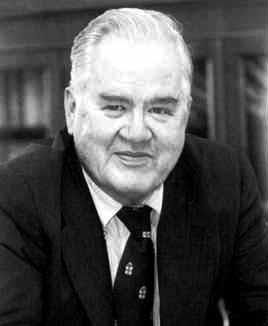John Tukey coined many terms that have passed into common use, such as bit (a shortening of binary digit) and software. Other terms he coined are well known within their niche: boxplot, ANOVA, rootogram, etc. Some of his terms, such as jackknife and vacuum cleaner, were not new words per se but common words he gave a technical meaning to.

Cepstrum is an anagram of spectrum. It involves an unusual use of power spectra, and is roughly analogous to making anagrams of a word. A related term, one we will get to shortly, is quefrency, an anagram of frequency. Some people pronounce the ‘c’ in cepstrum hard (like ‘k’) and some pronounce it soft (like ‘s’).

Let’s go back to an example from my post on guitar distortion. Here’s a note played with a fairly large amount of distortion:

And here is its power spectrum: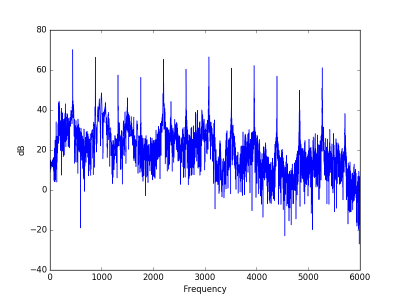There’s a lot going on in the spectrum, but the peaks are very regularly spaced. As I mentioned in the post on the sound of a leaf blower, this is the fingerprint of a sound with a definite pitch. Spikes in the spectrum alone don’t indicate a definite pitch if they are irregularly spaced.

The peaks are fairly periodic. How to you find periodic patterns in a signal? Fourier transform! But if you simply take the Fourier transform of a Fourier transform, you essentially get the original signal back. The key to the cepstrum is to do something else between the two Fourier transforms.

The cepstrum starts by taking the Fourier transform, then the magnitude, then the logarithm, and then the inverse Fourier transform.

When we take the magnitude, we throw away phase information, which we don’t need in this context. Taking the log of the magnitude is essentially what you do when you compute sound pressure level. Some define the cepstrum using the magnitude of the Fourier transform and some the magnitude squared. Squaring only introduces a multiple of 2 once we take logs, so it doesn’t effect the location of peaks, only their amplitude.

Taking the logarithm compresses the peaks, bringing them all into roughly the same range, making the sequence of peaks roughly periodic.

When we take the inverse Fourier transform, we now have something like a frequency, but inverted. This is what Tukey called quefrency.

Looking at the guitar power spectrum above, we see a sequence of peaks spaced 440 Hz apart. When we take the inverse Fourier transform of this, we’re looking at a sort of frequency of a frequency, what Tukey calls quefrency. The quefrency scale is inverted: sounds with a high frequency fundamental have overtones that are far apart on the frequency domain, so the sequence of the overtone peaks has low frequency.

Here’s the plot of the cepstrum for the guitar sample.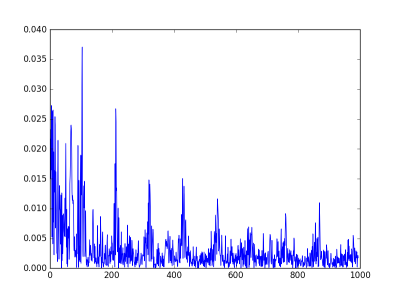There’s a big peak at 109 on the quefrency scale. The audio clip was recorded at 48000 samples per second, so the 109 on the quefrency scale corresponds to a frequency of 48000/109 = 440 Hz. The second peak is at quefrency 215, which corresponds to 48000/215 = 223 Hz. The second peak corresponds to the perceived pitch of the note, A3, and the first peak corresponds to its first harmonic, A4. (Remember the quefrency scale is inverted relative to the frequency scale.)

I cheated a little bit in the plot above. The very highest peaks are at 0. They are so large that they make it hard to see the peaks we’re most interested in. These low quefrency peaks correspond to very high frequency noise, near the edge of the audible spectrum or beyond.

# Electric guitar distortionThe other day I asked on Google+ if someone could make an audio clip for me and Dave Jacoby graciously volunteered. I wanted a simple chord on an electric guitar played with varying levels of distortion. Dave describes the process of making the recording as

Fender Telecaster -> EHX LPB clean boost -> Washburn Soloist Distortion (when engaged) -> Fender Frontman 25R amplifier -> iPhone

Let’s look at the Fourier spectrum at four places in the recording: single note and chord, clean and distorted. These are a 0:02, 0:08, 0:39, and 0:43.

## Power spectra

The first note, without distortion, has most of its spectrum concentrated at 220 Hz, the A below middle C.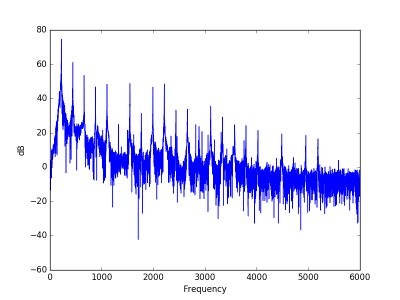The same note with distortion has a power spectrum that decays much slow, i.e. the sound has more high frequency components.Here’s the A major chord without distortion. Note that since the threshold of hearing is around 20 dB, most of the noise components are inaudible.Here’s the same chord with distortion. Notice there’s much more noise in the audible range.Update: See the next post an analysis of the loudness and sharpness of the audio samples in this post.

Photo via Brian Roberts CC

# Spectral flatness

White noise has a flat power spectrum. So a reasonable way to measure how close a sound is to being pure noise is to measure how flat its spectrum is.

Spectral flatness is defined as the ratio of the geometric mean to the arithmetic mean of a power spectrum.

The arithmetic mean of a sequence of n items is what you usually think of as a mean or average: add up all the items and divide by n.

The geometric mean of a sequence of n items is the nth root of their product. You could calculate this by taking the arithmetic mean of the logarithms of the items, then taking the exponential of the result. What if some items are negative? Since the power spectrum is the squared absolute value of the FFT, it can’t be negative.

So why should the ratio of the geometric mean to the arithmetic mean measure flatness? And why pure tones have “unflat” power spectra?

If a power spectrum were perfectly flat, i.e. constant, then its arithmetic and geometric means would be equal, so their ratio would be 1. Could the ratio ever be more than 1? No, because the geometric mean is always less than or equal to the arithmetic mean, with equality happening only for constant sequences.

In the continuous realm, the Fourier transform of a sine wave is a pair of delta functions. In the discrete realm, the FFT will be large at two points and small everywhere else. Why should a concentrated function have a small flatness score? If one or two of the components are 1’s and the rest are zeros, the geometric mean is zero. So the ratio of geometric and arithmetic means is zero. If you replace the zero entries with some small ε and take the limit as ε goes to zero, you get 0 flatness as well.

Sometimes flatness is measured on a logarithmic scale, so instead of running from 0 to 1, it would run from -∞ to 0.

Let’s compute the flatness of some examples. We’ll take a mixture of a 440 Hz sine wave and Gaussian white noise with varying proportions, from pure sine wave to pure noise. Here’s what the flatness looks like as a function of the proportions.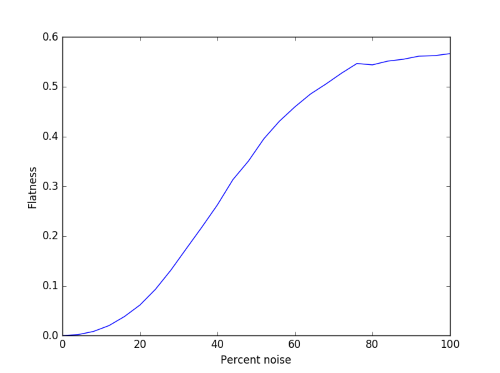The curve is fairly smooth, though there’s some simulation noise at the right end. This is because we’re working with finite samples.

Here’s what a couple of these signals would sound like. First 30% noise and 70% sine wave:

And now the other way around, 70% noise and 30% sine wave:

Why does the flatness of white noise max out somewhere between 0.5 and 0.6 rather than 1? White noise only has a flat spectrum in expectation. The expected value of the power spectrum at every frequency is the same, but that won’t be true of any particular sample.

RelatedPsychoacoustics

# How to create Green noise in Python

This is a follow-on to my previous post on green noise. Here we create green noise with Python by passing white noise through a Butterworth filter.

Green noise is in the middle of the audible spectrum (on the Bark scale), just where our hearing is most sensitive, analogous to the green light, the frequency where our eyes are most sensitive. See previous post for details, including an explanation of where the left and right cutoffs below come from.

Here’s the code:

from scipy.io.wavfile import write
from scipy.signal import buttord, butter, filtfilt
from scipy.stats import norm
from numpy import int16

def turn_green(signal, samp_rate):
# start and stop of green noise range
left = 1612 # Hz
right = 2919 # Hz

nyquist = (samp_rate/2)
left_pass  = 1.1*left/nyquist
left_stop  = 0.9*left/nyquist
right_pass = 0.9*right/nyquist
right_stop = 1.1*right/nyquist

(N, Wn) = buttord(wp=[left_pass, right_pass],
ws=[left_stop, right_stop],
gpass=2, gstop=30, analog=0)
(b, a) = butter(N, Wn, btype='band', analog=0, output='ba')
return filtfilt(b, a, signal)

def to_integer(signal):
# Take samples in [-1, 1] and scale to 16-bit integers,
# values between -2^15 and 2^15 - 1.
signal /= max(signal)
return int16(signal*(2**15 - 1))

N = 48000 # samples per second

white_noise= norm.rvs(0, 1, 3*N) # three seconds of audio
green = turn_green(white_noise, N)
write("green_noise.wav", N, to_integer(green))



And here’s what it sounds like:

Let’s look at the spectrum to see whether it looks right. We’ll use one second of the signal so the x-axis coincides with frequency when we plot the FFT.

from scipy.fftpack import fft

one_sec = green[0:N]
plt.plot(abs(fft(one_sec)))
plt.xlim((1500, 3000))
plt.show()


Here’s the output, concentrated between 1600 and 3000 Hz as expected: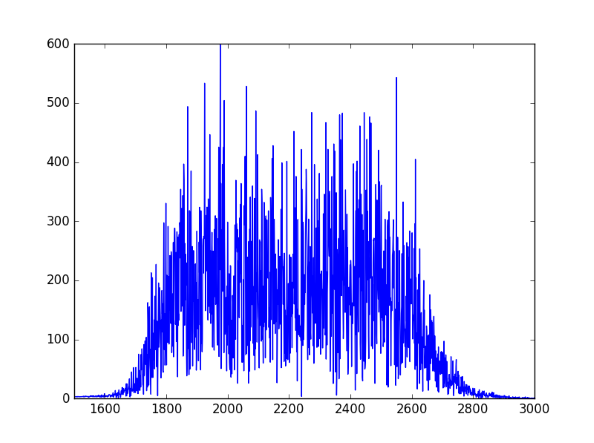# Beats: amplitude modulation in radios and musical instruments

What do tuning a guitar and tuning a radio have in common? Both are examples of beats or amplitude modulation.

## Examples

In an earlier post I wrote about how beats come up in vibrating systems, such as a mass and spring combination or an electric circuit. Here I look at examples from music and radio.

### Music

When two musical instruments play nearly the same note, they produce beats. The number of beats per second is the difference in the two frequencies. So if two flutes are playing an A, one playing at 440 Hz and one at 442 Hz, you’ll hear a pitch at 441 Hz that beats two times a second. Here’s a wave file of two pure sine waves at 440 Hz and 442 Hz.

As the players come closer to being in tune, the beats slow down. Sometimes you don’t have two instruments but two strings on the same instrument. Guitarists listen for beats to tell when two strings are playing the same note with the same pitch.

The same principle applies to AM radio. A message is transmitted by multiplying a carrier signal by the content you want to broadcast. The beats are the content. As we’ll see below, in some ways the musical example and the AM radio example are opposites. With tuning, we start with two sources and create beats. With AM radio, we start by creating beats, then see that we’ve created two sources, the sidebands of the signal.

## Mathematical explanation

Both examples above relate to the following trig identity:

cos(ab) + cos(a+b) = 2 cos a cos b

And because we’re looking at time-varying signals, slip in a factor of 2πt:

cos(2π(ab)t) + cos(2π(a+b)t) = 2 cos 2πat cos 2πbt

### Music

In the case of two pure tones, slightly out of tune, let a = 441 and b = 1. Playing an A 440 and an A 442 at the same time results in an A 441, twice as loud, with the amplitude going up and down like cos 2πt, i.e. oscillating two times a second. (Why two times and not just once? One beat for the maximum and one for the minimum of cos 2πt.)

It may be hard to hear beats because of complications we’ve glossed over. Musical instruments are never perfectly in phase, but more importantly they’re not pure tones. An oboe, for example, has strong components above the fundamental frequency. I used a flute in this example because although its tone is not simply a sine wave, it’s closer to a sine wave than other instruments, especially when playing higher notes. Also, guitarists often compare the harmonics of two strings. These are purer tones and so it’s easier to hear beats between them.

For the case of AM radio, read the equation above from right to left. Let a be the frequency of the carrier wave. For example if you’re broadcasting on AM station 700, this means 700 kHz, so a = 700,000. If this station were broadcasting a pure tone at 440 Hz, b would be 440. This would produce sidebands at 700,440 Hz and 699,560 Hz.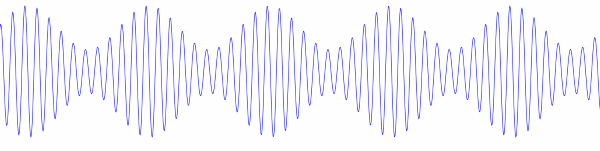In practice, however, the carrier is not multiplied by a signal like cos 2πbt but by 1 + m cos 2πbt where |m| < 1 to avoid over-modulation. Without this extra factor of 1 the signal would be 100% modulated; the envelope of the signal would pinch all the way down to zero. By including the factor of 1 and using a modulation index m less than 1, the signal looks more like the image above, with the envelope not pinching all the way down. (Over-modulation occurs when m > 1. Instead of the envelope pinching to zero, the upper and lower parts of the envelop cross.)

# Creating police siren sounds with frequency modulation

Yesterday I was looking into calculating fluctuation strength and playing around with some examples. Along the way I discovered how to create files that sound like police sirens. These are sounds with high fluctuation strength.The Python code below starts with a carrier wave at fc = 1500 Hz. Not surprisingly, this frequency is near where hearing is most sensitive. Then this signal is modulated with a signal with frequency fm. This frequency determines the frequency of the fluctuations.

The slower example produced by the code below sounds like a police siren. The faster example makes me think more of an ambulance or fire truck. Next time I hear an emergency vehicle I’ll pay more attention.

If you use a larger value of the modulation index β and a smaller value of the modulation frequency fm you can make a sound like someone tuning a radio, which is no coincidence.

Here are the output audio files in .wav format:

slow.wav

fast.wav

from scipy.io.wavfile import write
from numpy import arange, pi, sin, int16

def f(t, f_c, f_m, beta):
# t    = time
# f_c  = carrier frequency
# f_m  = modulation frequency
# beta = modulation index
return sin(2*pi*f_c*t - beta*sin(2*f_m*pi*t))

def to_integer(signal):
# Take samples in [-1, 1] and scale to 16-bit integers,
# values between -2^15 and 2^15 - 1.
return int16(signal*(2**15 - 1))

N = 48000 # samples per second
x = arange(3*N) # three seconds of audio

data = f(x/N, 1500, 2, 100)
write("slow.wav", N, to_integer(data))

data = f(x/N, 1500, 8, 100)
write("fast.wav", N, to_integer(data))


# Correlation of two sine waves

What is the correlation of two sine waves that differ in phase? The result itself is interesting, and the calculation along the way shows tricks to avoid calculating integrals.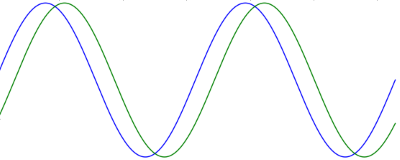The correlation of two periodic signals, f and g, iswhere the integral is over a period of the two functions. For functions known at discrete points this would be a sum rather than an integral, but in this case we have continuous signals so we integrate.

In our case the two functions are f(t) = sin(t) and g(t) = sin(t + φ) and the integrals are over [0, 2π]. Both functions have average value 0, so the μ terms go away.

We use a trig identity to expand the numerator:In our case θ1 = t and θ2 = t + φ and so the numerator becomesThe first part of the integral is integrating a constant (with respect to t) and so becomes the constant times the length of the integration range. The second part of the integral is zero because it is integrating a cosine over two periods.

Now for the denominator. Over a full period, sin2(t) and cos2(t) take on the same values, just shifted. So the integral of sin2(t) is half the integral of sin2(t) + cos2(t) = 1. Thereforeand the same argument shows that the integral of sin2(t + φ) is also π. So our correlation is simply cos φ: the correlation of two out-of-phase sine waves is the cosine of their phase difference. It may be a little surprising that it works out to be so simple, but the result makes sense. When φ = 0, or any multiple of 2π, the waves are identical and so the correlation should be 1. When φ = π, or an odd multiple of π, the two waves are perfectly out of phase, and so the correlation should be -1. In between these extremes the correlation oscillates, in fact it is a cosine.

# Energy in frequency modulated signals

In an earlier post we proved that if you modulate a cosine carrier by a sine signal you get a signal whose sideband amplitudes are given by Bessel functions. Specifically:When β = 0, we have the unmodulated carrier, cos(2π fct), on both sides. When β is positive but small, J0(β) is near 1, and so the frequency component corresponding to the carrier is only slightly diminished. Also, the sideband amplitudes, the values of Jn(β) for n ≠ 0, are small and decay rapidly as |n| increases. As β increases, the amplitude of the carrier component decreases, the sideband amplitudes increase, and the sidebands decay more slowly.

We can be much more precise: the energy in the modulated signal is the same as the energy in the unmodulated signal. As β increases, more energy transfers to the sidebands, but the total energy stays the same. This conservation of energy result applies to more complex signals than just pure sine waves, but it’s easier to demonstrate in the case of a simple signal.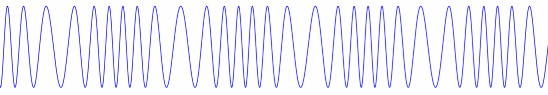## Proof

To prove the energy stays constant, we show that the sum of the squares of the coefficients of the cosine components is the same for the modulated and unmodulated signal.The unmodulated signal is just cos(2π fct), and so the only coefficient is 1. That means we have to proveThis is a well-known result. For example, it is equation 9.1.76 in Abramowitz and Stegun. We’ll show how to prove it from first principles. We’ll actually prove a more general result, the Newmann-Schläffi addition formula, then show our result follows easily from that.

Whittaker and Watson define the Bessel functions by their generating function:This means that when you expand the expression on the left as a power series in t, whatever is multiplied by tn is Jn(z) by definition. (There are other ways of defining the Bessel functions, but this way leads quickly to what we want to prove.)

We begin by factoring the Bessel generating function applied to zw.Next we expand both sides as power series.and look at the terms involving tn on both sides. On the left this is Jn(zw). On the right, we multiply two power series. We will get a term containing tn whenever we multiply terms tj and tk where j and k sum to n.The equation above is the Newmann-Schläffi addition formula.

### Sum of squared coefficients

To prove that the sum of the squared sideband coefficients is 1,  we apply the addition formula with n = 0, z = β, and w = -β.This proves what we were after:We used a couple facts in the last step that we haven’t discussed. The first was that J0(0) = 1. This follows from the generating function by setting z to 0 and taking the limit as t → 0. The second was that Jm(-β) = Jm(β). You can also see this from the generating function since negating z has the same effect as swapping t and 1/t.

# Quantifying Loudness

How do you quantify how loud a sound is? Sounds like a simple question, but it’s not.

## What is loudness?

It’s not hard to measure the physical intensity of a sound, but loudness is the perceived intensity of a sound. It is not a physical phenomena but a psychological phenomena.

Loudness is subjective, but not entirely so. There is general consensus regarding what it means for two sounds to be equally loud, and even for ratios, such as saying when one sound is twice as loud as the other. Loudness is quantifiable, but not easily so.

## What does loudness depend on?

Loudness depends on several properties of a sound, such as its frequency, bandwidth, and duration. Loudness must depend on frequency because sounds that are too low or too high have no loudness at all because we simply cannot hear them. But even with the range of audible frequencies, loudness varies quite a bit by pitch. The graph below, via Wikipedia, shows equal loudness contours. The blue lines are from work by Fletcher and Munson in 1937. The red lines are the revised curves per the ISO 226:2003 standard.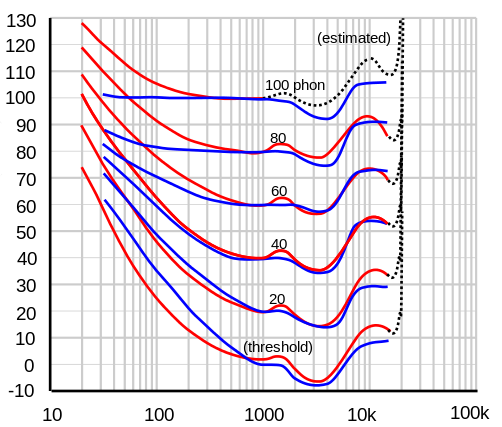The horizontal axis is frequency in Hz and the vertical axis is sound pressure level in decibels. The contour lines represent combinations of frequency and sound pressure level that are perceived to be equally loud. If a tuba and a flute sound equally loud, the sound pressure level coming from the tuba is much higher.

Notice that the curves are not parallel, They’re much closer together for low frequencies than for midrange frequencies, though they are roughly parallel for high frequencies. This means that if you recorded a piano, for example, playing each of its keys at equal loudness, the pitches wouldn’t sound equally loud unless you played the recording back at its original volume.

## Complexities and simplifications

As complicated as this is, it’s still a simplification. It is based on pure tones, simple sine waves. A single musical instrument, much less an orchestra or a jackhammer, are more complicated. Loudness is highly nonlinear, and so you cannot say that the loudness of two sounds is the sum of their individual loudnesses. A-weighting is a relatively simple way to convert sound pressure levels to loudness, but is only accurate for pure tones at fairly low loudness levels.

To simplify thing further, consider a single pure tone, a sine wave at 1 kHz. (This is almost two octaves above middle C. See details here.) Loudness level in phons is defined to match sound pressure level in decibels for a 1 kHz pure tone. So a sound has a loudness level of 40 phons, for example, if it is perceived to be as loud as a pure 1 hKz tone at 40 dB.

At 1 kHz, loudness increases by a factor of 2 for every 10 dB increase in sound pressure level. But because nothing is simple in psychoacoustics, even this is a simplification. It only holds for sounds with loudness level 40 dB or greater. A quiet room is around 40 phons, so the added complications below 40 phons may not be relevant in many applications.

A pure tone at 1 kHz and 20 dB sounds more than four times softer than the same tone at 40 dB. The definition of loudness level in phons still holds below 40 phons. An oboe has a loudness level of 20 phons if it has the same loudness as a sine wave with frequency 1 kHz and sound pressure level 20 dB. But an oboe at 30 phons will sound more than twice as loud as one at 20 phons.

Update: New blog post comparing guitar samples at the same sound pressure level but with differing loudness and sharpness.

## Summary

So where are we as far as calculating loudness? We’ve said a lot about what you can’t do, what complications have to be considered. But we’ve concluded this much: for a pure 1 kHz tone, the loudness in phons equals (by definition) the sound pressure level in decibels. And we’ve said how in principle you could define the loudness of other sounds: compare them to a 1 kHz tone that’s just as loud. We haven’t said how to compute this, only how you could determine it empirically.

In future posts I may write about how you do this using the ISO 532B standard or the newer ANSI S3.4-2007 standard.